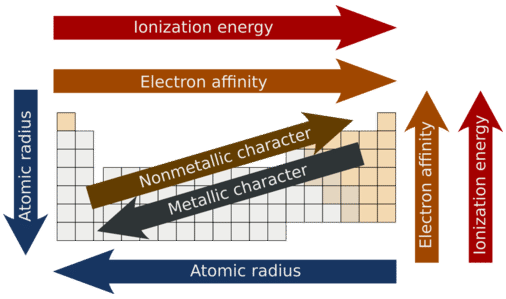# 2.8: Periodic Trends- Ionization Energy

Like many other animals, sheep travel in herds. The tendency is for each individual sheep to stay with the herd. However, a sheep may sometimes wander off, depending on how strong the attraction is for a particular food or water supply. At other times, a sheep may become frightened and run off. Whether a sheep chooses to stay with the herd or go its own way depends on the balance between attraction to the herd and attraction to an outside influence.

There is an ongoing tension between the electrons and protons in an atom. Reactivity of the atom depends in part on how easily the electrons can be removed from the atom. We can measure this quantity and use it to make predictions about the behavior of atoms.

## Ionization Energy

Ionization energy is the energy required to remove an electron from a specific atom. It is measured in $$\text{kJ/mol}$$, which is an energy unit, much like calories. The ionization energies associated with some elements are described in the table below. For any given atom, the outermost valence electrons will have lower ionization energies than the inner-shell kernel electrons. As more electrons are added to a nucleus, the outer electrons become shielded from the nucleus by the inner shell electrons. This is called electron shielding.

Table $$\PageIndex{1}$$: Ionization Energies $$\left( \text{kJ/mol} \right)$$ of the First 8 Elements
Element IE$$_1$$ IE$$_2$$ IE$$_3$$ IE$$_4$$ IE$$_5$$ IE$$_6$$
$$\ce{H}$$ 1312
$$\ce{He}$$ 2373 5251
$$\ce{Li}$$ 520 7300 11,815
$$\ce{Be}$$ 899 1757 14,850 21,005
$$\ce{B}$$ 801 2430 3660 25,000 32,820
$$\ce{C}$$ 1086 2350 4620 6220 38,000 47,261
$$\ce{N}$$ 1400 2860 4580 7500 9400 53,000
$$\ce{O}$$ 1314 3390 5300 7470 11,000 13,000

The following trend is seen when we plot the first ionization energies vs. atomic number for the main group elements:Figure $$\PageIndex{1}$$: Ionization energy and atomic number.

Moving from left to right across the periodic table, the ionization energy for an atom increases. We can explain this by considering the nuclear charge of the atom. The more protons in the nucleus, the stronger the attraction of the nucleus to electrons. This stronger attraction makes it more difficult to remove electrons.

Within a group, the ionization energy decreases as the size of the atom gets larger. On the graph, we see that the ionization energy increases as we go up the group to smaller atoms. In this situation, the first electron removed is farther from the nucleus as the atomic number (number of protons) increases. Being farther away from the positive attraction makes it easier for that electron to be pulled off.Figure $$\PageIndex{2}$$: Periodic trends.

## Summary

• Ionization energy refers to the amount of energy needed to remove an electron from an atom.
• Ionization energy decreases as we go down a group.
• Ionization energy increases from left to right across the periodic table.